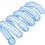# Big Number and Some Philosophizing

Here's a big number. It would be interesting to hear how big people think this is relative to other well-known big numbers, like Graham's number.

1) Define a "power tower" operation using the # symbol. Example:

$3 \text{\#} = 3^{3^3}$

So $N \text{\#}$ consists of $N$ elements in a power tower, in which each element is $N$.

2) Googol = G = $10^{100}$
3) Googolplex = $10^G$
4) $A = G \text{\#}$ (power tower a googolplex elements high, wherein each element is a googolplex)
5) Iteration:

$X_1 = A \text{\#} \\ X_2 = X_1 \text{\#} \\ X_3 = X_2 \text{\#} \\ \text{.} \\ \text{.} \\ \text{.} \\ X_A = X_{A-1} \text{\#}$

$X_A$ is the big number (subscript $A$ is the same quantity defined in Step 4)

Here are some more interesting questions:

a) Given $N$ characters within some generalized symbolic alphabet, what is the largest finite number that can be defined algorithmically using those $N$ characters, and how does the number relate to N?

b) How big a number could you define using a number of characters equivalent to those in the combined works of Shakespeare? Or does the size of the limiting number depend more on the conceptual potency of the method than on the number of characters used?

c) Is there a large-number-generating paradigm more potent than power towers and iteration?Note by Steven Chase
3 years, 3 months ago

This discussion board is a place to discuss our Daily Challenges and the math and science related to those challenges. Explanations are more than just a solution — they should explain the steps and thinking strategies that you used to obtain the solution. Comments should further the discussion of math and science.

When posting on Brilliant:

• Use the emojis to react to an explanation, whether you're congratulating a job well done , or just really confused .
• Ask specific questions about the challenge or the steps in somebody's explanation. Well-posed questions can add a lot to the discussion, but posting "I don't understand!" doesn't help anyone.
• Try to contribute something new to the discussion, whether it is an extension, generalization or other idea related to the challenge.

MarkdownAppears as
*italics* or _italics_ italics
**bold** or __bold__ bold
- bulleted- list
• bulleted
• list
1. numbered2. list
1. numbered
2. list
Note: you must add a full line of space before and after lists for them to show up correctly
paragraph 1paragraph 2

paragraph 1

paragraph 2

[example link](https://brilliant.org)example link
> This is a quote
This is a quote
    # I indented these lines
# 4 spaces, and now they show
# up as a code block.

print "hello world"
# I indented these lines
# 4 spaces, and now they show
# up as a code block.

print "hello world"
MathAppears as
Remember to wrap math in $$ ... $$ or $ ... $ to ensure proper formatting.
2 \times 3 $2 \times 3$
2^{34} $2^{34}$
a_{i-1} $a_{i-1}$
\frac{2}{3} $\frac{2}{3}$
\sqrt{2} $\sqrt{2}$
\sum_{i=1}^3 $\sum_{i=1}^3$
\sin \theta $\sin \theta$
\boxed{123} $\boxed{123}$

Sort by:

JAZF1 is also referred to as Tip27 and ZNF802 (molecular weight of coding protein is 27 KDa and its isoelectric point is 8.83). In fact, JAZF1 is a newly identified gene with unknown function. Some reports show that JAZF1 is the co-repressor for nuclear orphan receptor TAK1. Moreover, a recent study has shown that JAZF1 is related to prostate cancer, endometrial stromal tumor, but its function and specific pathogenit mechanisms of these diseases remain unclear.

https://www.creative-biogene.com/genesearch/JAZF1.html

- 1 year, 9 months ago